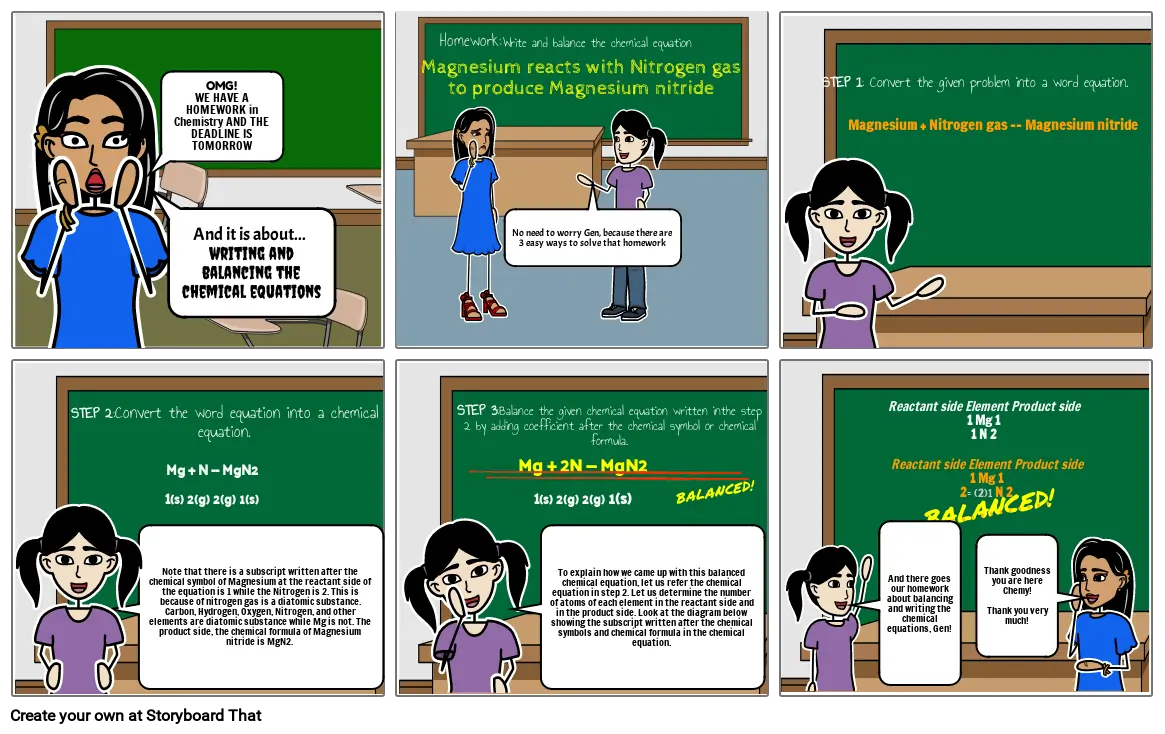# Balancing Equations

Create a Storyboard#### Storyboard Text

• OMG!WE HAVE A HOMEWORK in Chemistry AND THE DEADLINE IS TOMORROW
• And it is about... ﻿writing and balancing the chemical equations
• ﻿Magnesium reacts with Nitrogen gas to produce Magnesium nitride
• ﻿Homework:
• ﻿Write and balance the chemical equation
• No need to worry Gen, because there are 3 easy ways to solve that homework
• STEP 1: Convert the given problem into a word equation.
• Magnesium + Nitrogen gas -- Magnesium nitride
• Mg + N -- MgN21(s) 2(g) 2(g) 1(s)
• STEP 2:Convert the word equation into a chemical equation.
• Note that there is a subscript written after the chemical symbol of Magnesium at the reactant side of the equation is 1 while the Nitrogen is 2. This is because of nitrogen gas is a diatomic substance. Carbon, Hydrogen, Oxygen, Nitrogen, and other elements are diatomic substance while Mg is not. The product side, the chemical formula of Magnesium nitride is MgN2. ﻿
• Mg + 2N -- MgN21(s) 2(g) 2(g) 1(s)
• STEP 3:Balance the given chemical equation written inthe step 2 by adding coefficient after the chemical symbol or chemical formula.
• To explain how we came up with this balanced chemical equation, let us refer the chemical equation in step 2. Let us determine the number of atoms of each element in the reactant side and in the product side. Look at the diagram below showing the subscript written after the chemical symbols and chemical formula in the chemical equation.
• BALANCED!
• Reactant side Element Product side1 Mg 11 N 2
• Reactant side Element Product side 1 Mg 12= (2)1 N 2
• And there goes our homework about balancing and writing the chemical equations, Gen!
• BALANCED!
• Thank goodness you are here Chemy!Thank you very much!
Over 30 Million Storyboards Created